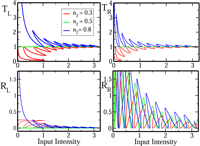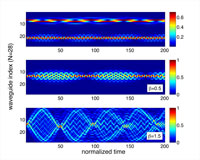August 2011
Mon Tue Wed Thu Fri Sat Sun
« Jul   Sep »
1234567
891011121314
15161718192021
22232425262728
293031

## Para-Grassmannian Coherent and Squeezed States for Pseudo-Hermitian q-Oscillator and their Entanglement

Yusef Maleki

In this parer, q-deformed oscillator for pseudo-Hermitian systems is investigated and pseudo-Hermitian appropriate coherent and squeezed states are studied. Also, some basic properties of these states is surveyed. The over-completeness property of the para-Grassmannian pseudo-Hermitian coherent states (PGPHCSs) examined, and also the stability of coherent and squeezed states discussed. The pseudo-Hermitian supercoherent states as the product of a pseudo-Hermitian bosonic coherent state and a para-Grassmannian pseudo-Hermitian coherent state introduced, and the method also developed to define pseudo-Hermitian supersqueezed states. It is also argued that, for q-oscillator algebra of $$k+1$$ degree of nilpotency based on the changed ladder operators, defined in here, we can obtain deformed $$SU_{q^2}(2)$$ and $$SU_{q^{2k}}(2)$$ and also $$SU_{q^{2k}}(1,1)$$. Moreover, the entanglement of multi-level para-Grassmannian pseudo-Hermitian coherent state will be considered. This is done by choosing an appropriate weight function, and integrating over tensor product of PGPHCSs.

http://arxiv.org/abs/1108.5005
Mathematical Physics (math-ph); High Energy Physics – Theory (hep-th); Quantum Physics (quant-ph)

## On the similarity of Sturm-Liouville operators with non-Hermitian boundary conditions to self-adjoint and normal operators

D. Krejcirik, P. Siegl, J. Zelezny

We consider one-dimensional Schroedinger-type operators in a bounded interval with non-self-adjoint Robin-type boundary conditions. It is well known that such operators are generically conjugate to normal operators via a similarity transformation. Motivated by recent interests in quasi-Hermitian Hamiltonians in quantum mechanics, we study properties of the transformations in detail. We show that they can be expressed as the sum of the identity and an integral Hilbert-Schmidt operator. In the case of parity and time reversal boundary conditions, we establish closed integral-type formulae for the similarity transformations, derive the similar self-adjoint operator and also find the associated “charge conjugation” operator, which plays the role of fundamental symmetry in a Krein-space reformulation of the problem.

http://arxiv.org/abs/1108.4946
Spectral Theory (math.SP); Mathematical Physics (math-ph); Functional Analysis (math.FA); Quantum Physics (quant-ph)

## Unidirectional Invisibility induced by PT-Symmetric Periodic StructuresZin Lin, Hamidreza Ramezani, Toni Eichelkraut, Tsampikos Kottos, Hui Cao, Demetrios N. Christodoulides

We show that parity-time (PT) symmetric Bragg periodic structures, near the spontaneous PT – symmetry breaking point, can act as unidirectional invisible media. In this regime, the re flection from one end is diminished while it is enhanced from the other. At the same time the transmission coefficient and phase, are indistinguishable from those expected in the absence of a grating. The phenomenon is robust even in the presence of Kerr non-linearities, and it can also eff?ectively suppress optical bistabilities.

http://arxiv.org/abs/1108.2493
Optics (physics.optics)

## Dynamics and disorder effects in waveguide lattices with localized eigenstatesHarsha Vemuri, Vaibhav Vavilala, Theja Bhamidipati, Yogesh N. Joglekar

Recently, waveguide lattices with non-uniform tunneling have been explored due to their myriad tunable properties, many of which arise from the extended nature of their eigenstates. Here, we investigate the dynamics, localization, and parity- and time-reversal-(PT) symmetry breaking in lattices with only localized eigenstates. We propose three families of tunneling profiles that lead to qualitatively different single-particle time evolution, and show that the effects of weak disorder contain signatures of the localized or extended nature of clean-lattice eigenstates. Our results suggest that waveguide lattices with only localized eigenstates will exhibit a wide array of phenomena that are absent in traditional systems.

http://arxiv.org/abs/1108.1402
Optics (physics.optics); Quantum Gases (cond-mat.quant-gas); Quantum Physics (quant-ph)

## Non-Hermitian multi-particle systems from complex root spaces

Andreas Fring, Monique Smith

We provide a general construction procedure for antilinearly invariant complex root spaces. The proposed method is generic and may be applied to any Weyl group allowing to take any element of the group as a starting point for the construction. Worked out examples for several specific Weyl groups are presented, focusing especially on those cases for which no solutions were found previously. When applied in the defining relations of models based on root systems this usually leads to non-Hermitian models, which are nonetheless physically viable in a self-consistent sense as they are antilinearly invariant by construction. We discuss new types of Calogero models based on these complex roots. In addition we propose an alternative construction leading to q-deformed roots. We employ the latter type of roots to formulate a new version of affine Toda field theories based on non-simply laced roots systems. These models exhibit on the classical level a strong-weak duality in the coupling constant equivalent to a Lie algebraic duality, which is known for the quantum version of the undeformed case.

http://arxiv.org/abs/1108.1719
High Energy Physics – Theory (hep-th); Mathematical Physics (math-ph); Quantum Physics (quant-ph)

## Decays of degeneracies in PT-symmetric ring-shaped lattices

Miloslav ZnojilNon-Hermitian ring-shaped discrete lattices share the appeal with their more popular linear predecessors. Their dynamics controlled by the nearest-neighbor interaction is equally phenomenologically interesting. In comparison, the innovative nontriviality of their topology may be expected to lead to new spectral effects. Some of them are studied here via solvable examples. Main attention is paid to the perturbation-caused removals of spectral degeneracy at exceptional points.

http://arxiv.org/abs/1108.0620
Quantum Physics (quant-ph); Mathematical Physics (math-ph)

## Resolutions of identity for some non-Hermitian Hamiltonians II: proofs

A.V. Sokolov

This part is a continuation of the Part I where we built resolutions of identity for certain non-Hermitian Hamiltonians constructed from biorthogonal sets of their eigen- and associated functions for the spectral problem defined on entire axis. Non-Hermitian Hamiltonians under consideration were taken with continuous spectrum and the following cases were examined: an exceptional point situated on a boundary of continuous spectrum and an exceptional point situated inside of continuous spectrum. In the present work the rigorous proofs are given for the resolutions of identity in both cases.

http://arxiv.org/abs/1107.5916
Mathematical Physics (math-ph); High Energy Physics – Theory (hep-th); Nuclear Theory (nucl-th); Quantum Physics (quant-ph)

## Resolutions of identity for some non-Hermitian Hamiltonians I: exceptional point in continuous spectrum

A.A. Andrianov, A.V. Sokolov

Resolutions of identity for certain non-Hermitian Hamiltonians constructed from biorthogonal sets of their eigen- and associated functions are given for the spectral problem defined on entire axis. Non-Hermitian Hamiltonians under consideration are taken with continuous spectrum and the following peculiarities are investigated: (1) the case when there is an exceptional point situated on a boundary of continuous spectrum; (2) the case when there is an exceptional point situated inside of continuous spectrum. The reductions of the derived resolutions of identity under narrowing of the classes of employed test functions are revealed. It is shown that in the case (1) some of associated functions included into the resolution of identity are normalizable and some of them may be not and in the case (2) the bounded associated function corresponding to the exceptional point does not belong to the physical state space. Spectral properties of a SUSY partner Hamiltonian for the Hamiltonian with an exceptional point are examined.

http://arxiv.org/abs/1107.5911
Mathematical Physics (math-ph); High Energy Physics – Theory (hep-th); Nuclear Theory (nucl-th); Quantum Physics (quant-ph)